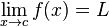## Limit Calculator

Limits are essential to calculus. The calculator will computes the limit of given function. In mathematics, a limit is the value that a function "approaches" as the input.

### The limit Formula:For Examples

limx→3(5x-6) = 9, The limit of -6 + 5x approaches 3 is 9.

limx→3(x3-6) = 21, The limit of -6 + x3 approaches 3 is 21.

Thinkcalculator.com provides you helpful and handy calculator resources.